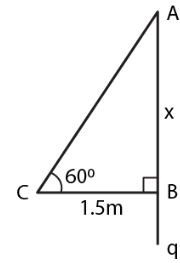Guru

# A ladder is placed against a wall such that it reaches the top of the wall. The foot of the ladder is 1.5 metres away from the wall and the ladder is inclined at an angle of 600 with the ground. Find the height of the wall.

• 0

sir this is the question from the book -ML aggarwal( avichal publication) class 10th , chapter20 , heights and distances
we have information about the questionn that A ladder is placed against a wall on the condition that  it reaches the top of the wall.
The foot of the ladder is 1.5 metres away from the wall and the ladder is inclined at an angle of 600 with the ground.
now how can we Find the height of the wall? explain in detail.

question no 3 , heights and distances , ICSE board

Share

1. Consider AB as the wall and AC as the ladder whose foot C is 1.5 m away from B

Take AB = x m and angle of inclination is 600We know that

tan θ = AB/CB

Substituting the values

tan 600 = x/1.5

So we get

√3 = x/1.5

By cross multiplication

x = √3 × 1.5 = 1.732 × 1.5

x = 2.5980 = 2.6

Hence, the height of wall is 2.6 m

• 0